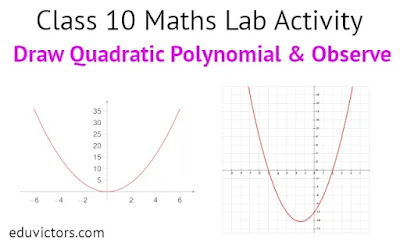# CBSE Class 10 Maths Lab Activity - Draw Quadratic Polynomial and Observe

OBJECTIVE: To draw the graph of a quadratic polynomial and observe.

(i) The shape of the curve when the coefficient of x² is positive.

(ii) The shape of the curve when the coefficient of x² is negative.

(iii) Its number of zeroes.

MATERIAL REQUIRED

Cardboard, graph paper, ruler, pencil, eraser, pen, adhesive.

STEPS OF CONSTRUCTION

1. Take cardboard of a convenient size and paste a graph paper on it.

2. Consider a quadratic polynomial f(x) = ax² + bx + c

3. Two cases arise:

(i) a > 0 (ii) a < 0

4. Find the ordered pairs (x, f (x)) for different values of x.

5. Plot these ordered pairs in the cartesian plane

6. Join the plotted points by a free hand curve. Based on polynomial used, you may have graphs as shown in following figures:

DEMONSTRATION

1. The shape of the curve obtained in each case is a parabola.

2. Parabola opens upward when coefficient of x² is positive.

3. It opens downward when coefficient of x² is negative.

4. Maximum number of zeroes which a quadratic polynomial can have is 2.

OBSERVATION

1. Parabola in Fig. 1 opens ______

2. Parabola in Fig. 2 opens _______

3. In Fig. 1, parabola intersects x-axis at ______ point(s).

4. Number of zeroes of the given polynomial is ________.

5. Parabola in Fig. 2 intersects x-axis at ______ point(s).

6. Number of zeroes of the given polynomial is ______.

7. Parabola in Fig.3 intersects x-axis at ______ point(s).

8. Number of zeroes of the given polynomial is _______.

9. Maximum number of zeroes which a quadratic polynomial can have is __________.

APPLICATION

This activity helps in:

1. understanding the geometrical representation of a quadratic polynomial

2. finding the number of zeroes of a quadratic polynomial

A Sample project is shown here.